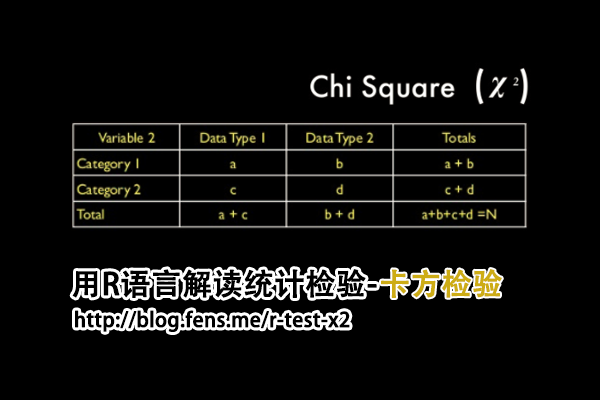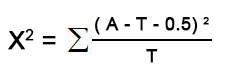• Posts tagged "四格表法"

# Blog Archives

## 用R语言解读统计检验-卡方检验

R的极客理想系列文章，涵盖了R的思想，使用，工具，创新等的一系列要点，以我个人的学习和体验去诠释R的强大。

R语言作为统计学一门语言，一直在小众领域闪耀着光芒。直到大数据的爆发，R语言变成了一门炙手可热的数据分析的利器。随着越来越多的工程背景的人的加入，R语言的社区在迅速扩大成长。现在已不仅仅是统计领域，教育，银行，电商，互联网….都在使用R语言。

• 张丹(Conan), 程序员/Quant: Java,R,Nodejs
• blog: http://blog.fens.me
• email: bsspirit@gmail.com

http://blog.fens.me/r-test-x21. 卡方检验介绍
2. 数据集
3. 四格表法推导过程
4. 程序实现

## 1. 卡方检验介绍

• χ2，为统计量，用于衡量实际值与理论值的差异程度。
• A，为实际值
• T，为理论值
• 自由度，df = n-1

## 2. 数据集

• Win10 64bit
• R: 3.6.1 x86_64-w64-mingw32/x64 b4bit

``````
> N=100                 # 抛硬币100次
> set.seed(1)           # 随机种子
> coin<-sample(x=c(0,1), prob=c(0.5,0.5),size = N, replace = TRUE)  # 模拟抛硬币
> coin                  # 打印结果
 1 1 0 0 1 0 0 0 0 1 1 1 0 1 0 1 0 0 1 0 0 1 0 1 1 1 1 1 0 1 1 0 1 1 0 0 0 1 0 1 0 0 0 0
 0 0 1 1 0 0 1 0 1 1 1 1 1 0 0 1 0 1 1 1 0 1 1 0 1 0 1 0 1 1 1 0 0 1 0 0 1 0 1 1 0 1 0 1
 1 1 1 1 0 0 0 0 1 1 0 0
``````

``````
> table(coin)  # 统计0和1的出现次数
coin
0  1
48 52
``````

``````
> cnt <- cumsum(coin)  # 积累求和，正面1出现的次数
> n<-1:N               # 次数向量
> rate<- cnt/1:N       # 累积的正面出现概率
> plot(n,rate,type="o",log="x",   # 画图，x轴做log变型
+      xlim=c(1,N), ylim=c(0.0,1.0), cex.axis=1.5,
``````

## 3. 四格表法推导过程

 正面 反面 合计 观察次数 52 48 100 期望次数 50 50 100 合计 102 98 200

 正面 反面 合计 观察次数 100*0.51 =51 100*(1-0.51) = 49 100 期望次数 100*0.51 =51 100*(1-0.51) = 49 100 合计 102 98 200

``````
X^2 = (52- 51)^2 / 51 + (48 - 49)^2 / 49 + (50 - 51)^2 / 51 + (50 - 49)^2 / 49 = 0.08003201
``````

1. 所有的理论数T≥5并且总样本量n≥40，用Pearson卡方进行检验.
2. 如果理论数T＜5但T≥1,并且n≥40，用连续性校正的卡方进行检验.
3. 如果有理论数T＜1或n＜40，则用Fisher’s检验.``````
X^2 = (abs(52- 51)-0.5)^2 / 51 + (abs(48 - 49)-0.5)^2 / 49 + (abs(50 - 51)-0.5)^2 / 51 + (abs(50 - 49)-0.5)^2 / 49
= 0.020008
``````

## 4. 程序实现

``````
# 合并观测值和期望值，形成矩阵
> s<-c(table(coin),c(50,50))
> x <- matrix(s, ncol = 2, byrow=TRUE)
> x
[,1] [,2]
[1,]   48   52
[2,]   50   50

# 卡方检验
> chisq.test(x)

Pearson's Chi-squared test with Yates' continuity correction

data:  x
X-squared = 0.020008, df = 1, p-value = 0.8875
``````

• H0:抛硬币出现正面和反面的概率都是50%，与次数无关
• X-squared统计量：0.020008
• df，自由度，1
• p-value值：0.8875

``````
# 查看观察值
> chi\$observed
[,1] [,2]
[1,]   48   52
[2,]   50   50

# 查看期望值
> chi\$expected
[,1] [,2]
[1,]   49   51
[2,]   49   51

# 残差
> chi\$residuals
[,1]      [,2]
[1,] -0.1428571  0.140028
[2,]  0.1428571 -0.140028
``````

``````
# 手动计算X^2值
> sr <- rowSums(x)
> sc <- colSums(x)
> n <- sum(x)
> E <- outer(sr, sc, "*")/n;E
[,1] [,2]
[1,]   49   51
[2,]   49   51
> x2 <- sum((abs(x - E) - 0.5)^2/E);x2
 0.020008

# 手动计算P值
> pchisq(x2, 1, lower.tail = FALSE)
 0.8875147
``````

http://blog.fens.me/r-test-x2